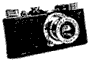Archived posting to the Leica Users Group, 1997/04/01

[Author Prev] [Author Next] [Thread Prev] [Thread Next] [Author Index] [Topic Index] [Home] [Search]

Subject: Picture quality and monitors (no Leica content!)
From: Jack Milton <jmilton@agate.net>
Date: Tue, 1 Apr 97 22:17:43 -0500

``` I went through the problem of poor picture quality on some monitors =
when we were setting up our web site at the newspaper I work at. =
Here's some of what I learned.

Most of the problems were traced to poor quality monitors, or poor =
quality video being sent to PC monitors. Macintosh has better video =
right out of the box =8B more colors (bit depth), more resolution, =
etc. This is *not* to say that good quality video cannot be had on =
PCs, just that many entry-level PCs do not have enough video-RAM, or =
good video cards to deliver good video to their monitors. Also many =
of the PC monitors were not of the best quality. This is changing, =
especially with newer and more expensive PC systems.
That being said, there are some things that can be done to assure =
that quality is there (in the images) to begin with.

Make sure your monitor is calibrated. Calibration of monitors to =
output is a never-ending task and learning process. Be mighty =
suspicious of so-called experts who claim to be able to calibrate all =
your monitors. I've not seen it happen yet.
Adobe Photoshop (for Mac, anyway) comes with a control panel named =
"Gamma." It's in the Goodies: Calibration folder. Drag it to your =
System folder, restart, and read the section in the Photoshop manual =
Although not perfectly precise, it works very well, and gives you a =
starting point that assures some level of uniformity in your images' =
color balance and density.If you have set it up properly, you can be =
(almost) sure that any poor quality viewing experiences can be traced =
to the user's monitor, and not to yours.

Save your continuous tone images (photos) as JPEG for your Web =
pages. JPEGs can look better than GIFs, which are limited to 256 =
colors. JPEGs will be displayed at the user's monitor's bit depth, up =
to 24-bit color (millions of colors), while GIFs are only displayed =
at up to 8-bit color (256 colors). JPEG at "Medium" quality =8B this =
give a small file size, with plenty of quality for viewing on a =
monitor. GIFs tend to get loaded onto the page a bit faster even =
though the JPEG'd image can be a smaller file size, but the time is =
negligible.
If you'd like to display a large, screen-filling image, consider =
making it a link from a smaller version of the same image.

1.0, Threshold 0 for the photos on our site. They might even be able =
to be sharpened further, but this works well for the size image we =
use.

Hope some of this helps. I am not a Web, or a computer expert, just =
a photographer who had to (and wanted to) learn a little about both. =
There is a link to the newspaper's site from my Web page.

- -Jack Milton
=3D=3D=3D=3D=3D=3D=3D=3D=3D=3D=3D=3D=3D=3D=3D=3D=3D=3D=3D=3D=3D=3D=3D=
=3D=3D=3D=3D=3D=3D=3D=3D=3D=3D=3D=3D=3D=3D=3D=3D=3D=3D=3D=3D=3D=3D=3D=
=3D=3D=3D=3D=3D=3D=3D=3D=3D=3D=3D=3D=3D=3D=3D=3D=3D=3D=3D=3D=3D=3D=3D=
=3D=3D=3D=3D
Many hands make light work. Too many cooks spoil the broth. I'm =
confused.
=3D=3D=3D=3D=3D=3D=3D=3D=3D=3D=3D=3D=3D=3D=3D=3D=3D=3D=3D=3D=3D=3D=3D=
=3D=3D=3D=3D=3D=3D=3D=3D=3D=3D=3D=3D=3D=3D=3D=3D=3D=3D=3D=3D=3D=3D=3D=
=3D=3D=3D=3D=3D=3D=3D=3D=3D=3D=3D=3D=3D=3D=3D=3D=3D=3D=3D=3D=3D=3D=3D=
=3D=3D=3D=3D
<http://www.agate.net/~jmilton/index.html>
```